Courses

# Notes | EduRev

## JEE Revision Notes

Created by: Learners Habitat

## JEE : Notes | EduRev

The document Notes | EduRev is a part of the JEE Course JEE Revision Notes.
All you need of JEE at this link: JEE

Gravitation:-

• Kepler’s first law (law of elliptical orbit):- A planet moves round the sun in an elliptical orbit with sun situated at one of its foci.
• Kepler’s second law (law of areal velocities):- A planet moves round the sun in such a way that its areal velocity is constant.
• Kepler’s third law (law of time period):- A planet moves round the sun in such a way that the square of its period is proportional to the cube of semi major axis of its elliptical orbit.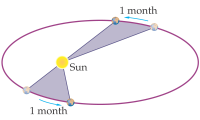T∝ R3
Here R is the radius of orbit.
T2 = (4π2/GM)R 3
• Newton’s law of gravitation:-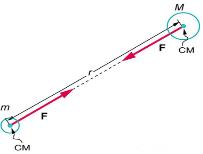Every particle of matter in this universe attracts every other particle with a forcer which varies directly as the product of masses of two particles and inversely as the square of the distance between them.
F= GMm/r2
Here, G is universal gravitational constant. G = 6.67 ´10 -11 Nm2 / kg2
• Dimensional formula of G: G = Fr2/Mm =[MLT-2][L2]/[M2] = [M-1L3T-2]
• Acceleration due to gravity (g):- g = GM/R2
• Variation of g with altitude:- g' = g(1- 2h/R),  if h<<R. Here R is the radius of earth and h is the height of the body above the surface of earth.
• Variation of g with depth:- g' = g(1- d/R). Here g' be the value of acceleration due to gravity at the depth d.
• Variation with latitude:-
At poles:- θ = 90°, g' = g
At equator:- θ = 0°, g' = g (1-ω2R/g)
Here ω is the angular velocity.
• As g = GMe/Re2 , therefore gpole > gequator
• Gravitational Mass:- m = FR2/GM
• Gravitational field intensity:-
E = F/m
= GM/r2
• Weight:- W= mg
• Gravitational intensity on the surface of earth (Es):-
Es = 4/3 (πRρG)
Here R is the radius of earth, ρ is the density of earth and G is the gravitational constant.
• Gravitational potential energy (U):- U = -GMm/r
(a) Two particles: U = -Gm1m2/r
(b) hree particles: U = -Gm1m2/r12 – Gm1m3/r13 – Gm2m3/r23
• Gravitational potential (V):- V(r) =  -GM/r
At surface of earth,
Vs=  -GM/R
Here R is the radius of earth.
• Escape velocity (ve):-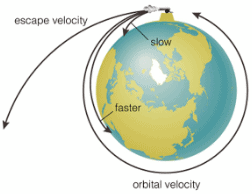It is defined as the least velocity with which a body must be projected vertically upward   in order that it may just escape the gravitational pull of earth.
ve = √2GM/R
or, ve = √2gR = √gD
Here R is the radius of earth and D is the diameter of the earth.
• Escape velocity (ve) in terms of earth’s density:- ve = R√8πGρ/3
• Orbital velocity (v0):-
v0 = √GM/r
If a satellite  of mass m revolves in a circular orbit around the earth of radius R and h be the height of the satellite above the surface of the earth, then,
r = R+h
So, v0 = √MG/R+h = R√g/R+h
In the case of satellite, orbiting very close to the surface of earth, then orbital velocity will be,
v= √gR
• Relation between escape velocity ve and orbital velocity v0 :- v0= ve/√2  (if h<<R)
• Time period of Satellite:- Time period of a satellite is the time taken by the satellite to complete one revolution around the earth.
T = 2π√(R+h)3/GM = (2π/R)√(R+h)3/g
If h<<R, T = 2π√R/g
• Height of satellite:- h = [gR2T2/4π2]1/3 – R
• Energy of satellite:-
Kinetic energy, K = ½ mv02 = ½ (GMm/r)
Potential energy, U = - GMm/r
Total energy, E = K+U
= ½ (GMm/r) + (- GMm/r)
= -½ (GMm/r)
• Gravitational force in terms of potential energy:- F = – (dU/dR)
• Acceleration on moon:-
gm = GMm/Rm2 = 1/6 gearth
Here Mm is the mass of moon and Rm is the radius of moon.
• Gravitational field:-
(a) Inside:-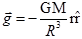(b) Outside:-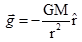• GRAVITATIONAL POTENTIAL & FIELD DUE TO VARIOUS OBJECTS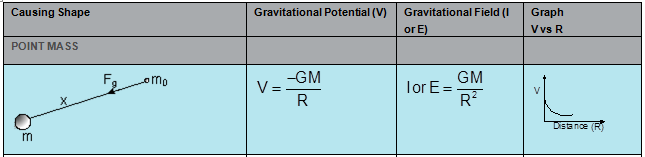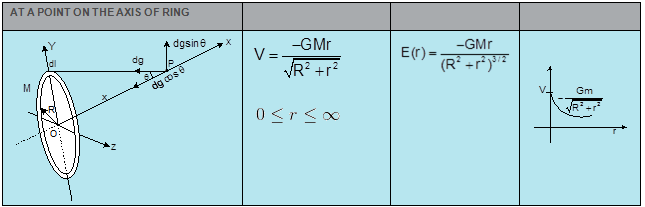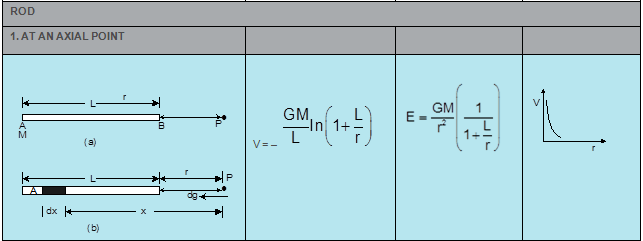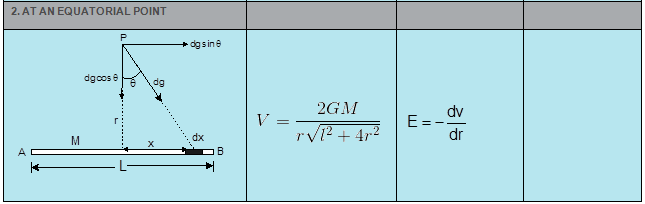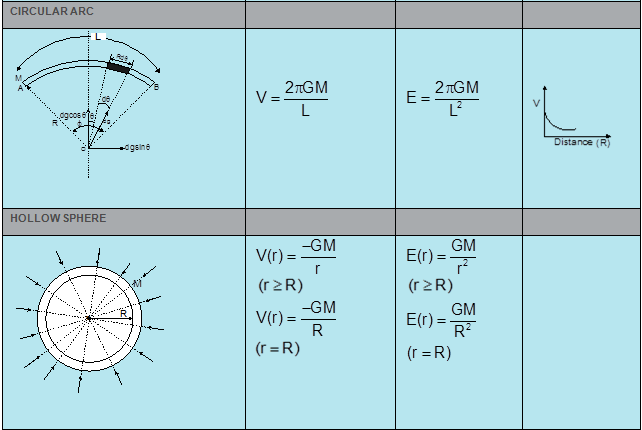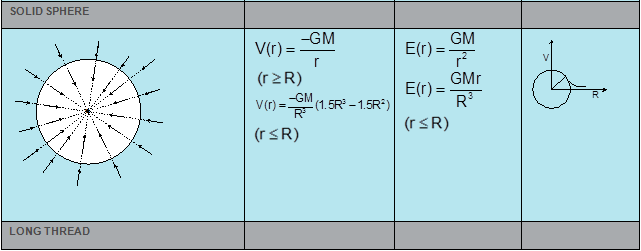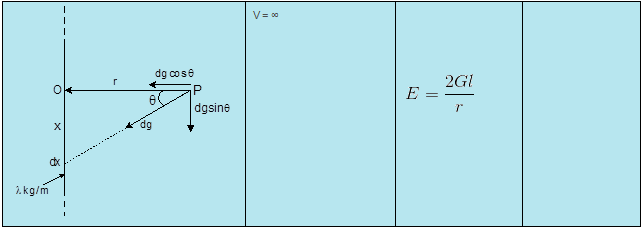Projectile:-

• Projectile fired at angle α  with the horizontal:- If a particle having initial speed u is projected at an angle α (angle of projection) with x-axis, then,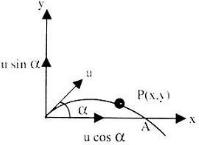Time of Ascent, t = (u sinα)/g
Total time of Flight, T = (2u sinα)/g
Horizontal Range, R = u2sin2α/g
Maximum Height, H = u2sin2α/2g
Equation of trajectory, y = xtanα-(gx2/2u2cos2α)
Instantaneous velocity, V=√(u2+g2t2-2ugt sinα)
and
β = tan-1(usinα-gt/ucosα)
• Projectile fired horizontally from a certain height:-
Equation of trajectory: x2 = (2u2/g)y
Time of descent (timer taken by the projectile to come down to the surface of earth), T = √2h/g
Horizontal Range, H = u√2h/g. Here u is the initial velocity of the body in horizontal direction.

Instantaneous velocity:-
V=√u2+g2t2
If β be the angle which V makes with the horizontal, then,
β = tan-1(-gt/u)
• Projectile fired at angle α with the vertical:-
Time of Ascent, t = (u cosα)/g
Total time of Flight, T = (2u cosα)/g
Horizontal Range, R = u2sin2α/g
Maximum Height, H = u2cos2α/2g
Equation of trajectory, y = x cotα-(gx2/2u2sin2α)
Instantaneous velocity, V=√(u2+g2t2-2ugt cosα) and β = tan-1(ucosα-gt/usinα)
• Projectile fired from the base of an inclined plane:-
Horizontal Range, R = 2u2 cos(α+β) sinβ/gcos2α
Time of flight, T = 2u sinβ/ gcosα
Here, α+β=θ
Offer running on EduRev: Apply code STAYHOME200 to get INR 200 off on our premium plan EduRev Infinity!

233 docs

,

,

,

,

,

,

,

,

,

,

,

,

,

,

,

,

,

,

,

,

,

;